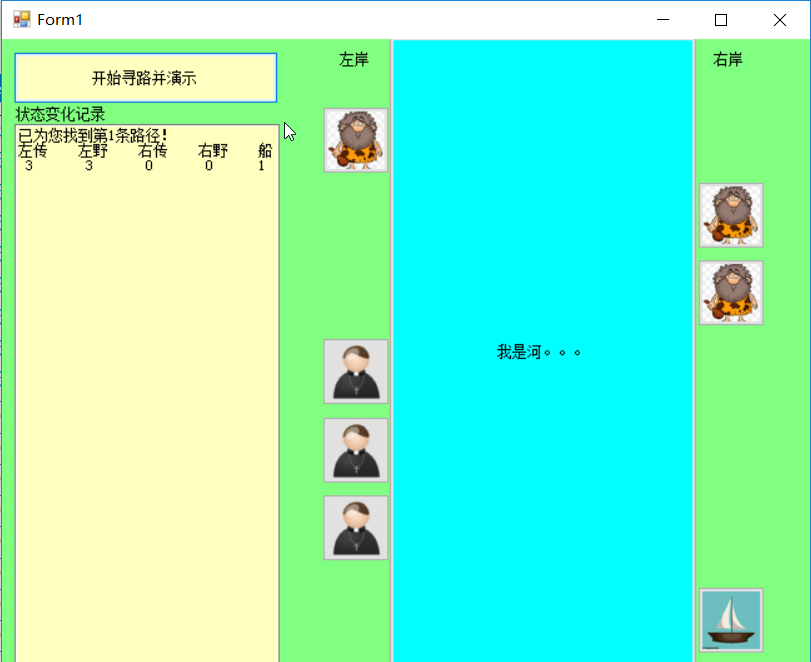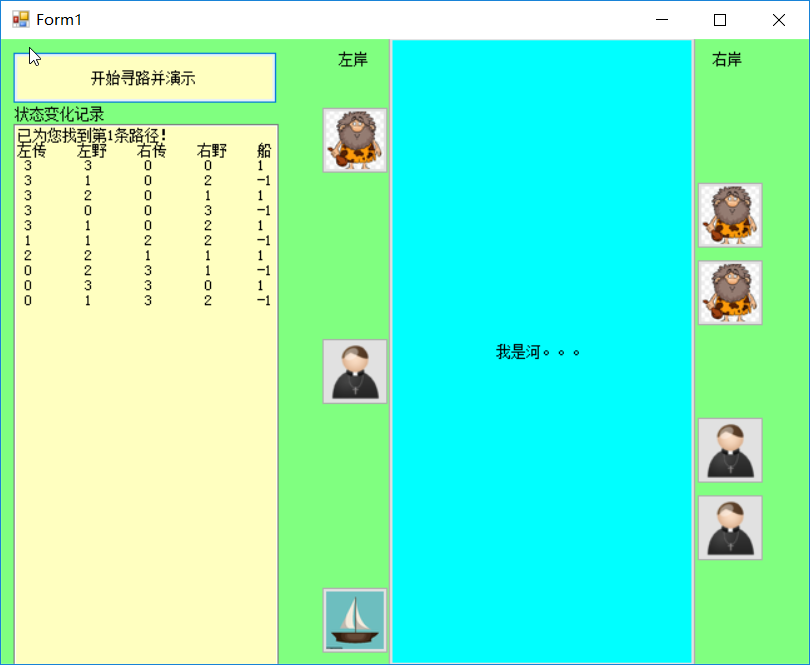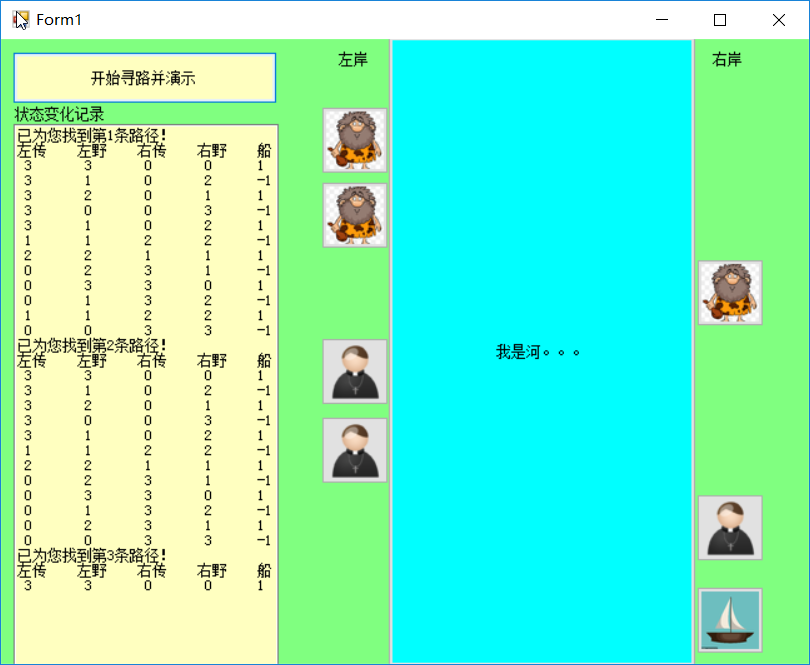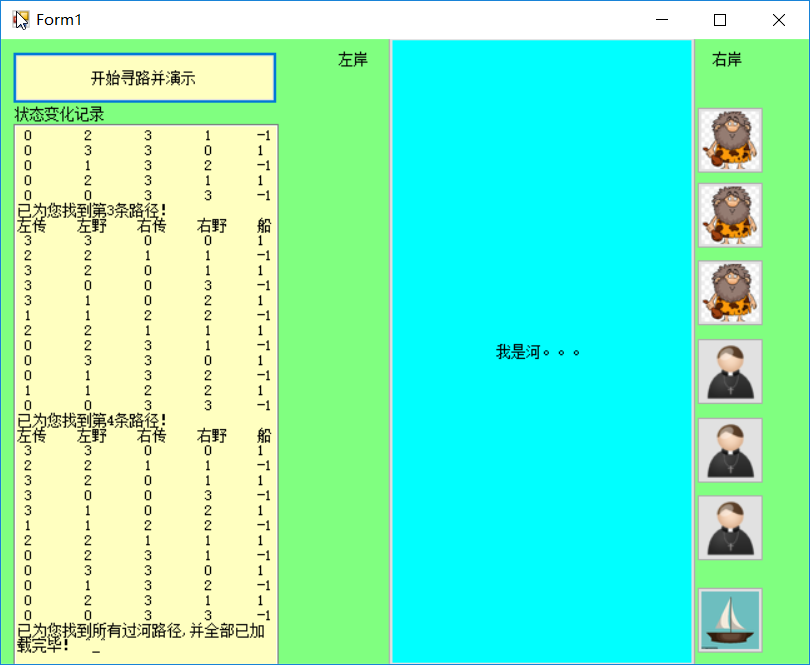# C#实现野人与传教士过河动画演示

## 野人与传教士过河问题

（1）渡2传教士
（2）渡2野人
（3）渡1野人1传教士
（4）渡1传教士
（5）渡1野人

（1）在此，对于C#在动画实现部分进行一些说明总结。初期，我是想通过在for循环打印信息的时候实现按钮的隐藏和显示来实现动画演示的，但是事实上并不是我想象的那么简单的，通过许多方法实践后，发现在for循环里面无法对按钮的能否可见进行操作，而需要等待for循环完成以后，设定的部分按钮才会显示出来。这也就是说不能通过该方法来实现动画演示了。后来我想到直接移动按钮的方法，发现该方法可以在for循环当中进行操作，于是，实现了动画演示的第一步。但是问题在于，我怎么控制那个按钮跳过河（左移），跳过河的按钮不能继续往前跳（左移），而只能往回跳（右移），但是指定按钮来执行跳过河，这样就会出现一个问题，例如，第一次，指定野人按钮1，和野人按钮2跳过河，之后，那个按钮跳回来呢？（程序上实现不了）就算强行指定一个，跳回来以后，我需要再次执行两个野人按钮跳过河，同样是执行刚才的代码，但是，刚才是指定的是野人按钮1，和野人按钮2，而现在应该是回来的野人按钮和野人按钮3过河。所以在程序上如果指定那个人物按钮跳过河，是实现不了动画演示的。
（2）现在，介绍程序是如何解决上述问题的。首先，我将所有状态按钮分左岸传教士和野人，右岸传教士和野人共计四组，分别添加进四个集合，表示不同岸的不同人物。 将左岸的集合大小直接复制给变量Ly（左岸的野人）和Lc（左岸的传教士），而将右岸的集合大小减4（3-4）以后赋值给Ry（右岸的野人）和Rc（右岸的传教士），也可以直接复制-1。这种思想类似于存在一个指向（最近写程序频繁受益于该思想），该指向在初始下指向左岸集合的末尾元素，而右岸没有人，所以指向-1,当发生过河事件的时候，利用Ly、Lc、Ry、Rc来改变指向位置，例如在初始状态下，过河连个野人，按照程序当中设计的结构，Ly会被连续两次执行减一操作，二Ry会连续两次执行加一操作。通过这种思想，我只用判断岸上是否有人存在，并且按照已经产生的正确的过程状态来进行按钮移动，也就不会出错，详见程序（表达能力欠缺，可能未表达出正确思想，可参照程序理解）。

//左岸到右岸
if (list[i].getBoat_location() == 1)
{
cc.Left += 300;
if (Math.Abs(list[i+1].getLeft_c()-list[i].getLeft_c()) >= 1)
{
Lc--;
Rc++;
Left_c[Lc].Left += 300;
if (Math.Abs(list[i + 1].getLeft_c() - list[i].getLeft_c()) >= 2)
{
Lc--;
Rc++;
Left_c[Lc].Left += 300;
if (Math.Abs(list[i + 1].getLeft_c() - list[i].getLeft_c()) == 3)
{
Lc--;
Rc++;
Left_c[Lc].Left += 300;
}
}
}
if (Math.Abs(list[i+1].getLeft_y()-list[i].getLeft_y()) >= 1)
{
Ly--;
Ry++;
Left_y[Ly].Left += 300;
if (Math.Abs(list[i + 1].getLeft_y() - list[i].getLeft_y()) >= 2)
{
Ly--;
Ry++;
Left_y[Ly].Left += 300;
if (Math.Abs(list[i + 1].getLeft_y() - list[i].getLeft_y()) == 3)
{
Ly--;
Ry++;
Left_y[Ly].Left += 300;
}
}
}
}using System;
using System.Collections.Generic;
using System.ComponentModel;
using System.Data;
using System.Drawing;
using System.Linq;
using System.Text;
using System.Windows.Forms;
//定义过河状态类
namespace C_Y_H
{
public partial class Form1 : Form
{
public Form1()
{
InitializeComponent();
}
List<RiverSide> list = new List<RiverSide>();
List<Control> Left_c = new List<Control>();
List<Control> Left_y = new List<Control>();
List<Control> Right_c = new List<Control>();
List<Control> Right_y = new List<Control>();
int  passNum = 0;
int Ly;
int Ry;
int Lc;
int Rc;
public int handle(RiverSide boat)
{
//是否达到目标转态
if (boat.getRight_c() == 3 && boat.getRight_y() == 3)
{
passNum++;
textBox1.AppendText("\n\n已为您找到第" + passNum + "条路径！\n\n");
textBox1.AppendText("\n\n左传" + "\t" + "左野" + "\t" + "右传" + "\t" + "右野" + "\t" + "船\n\n");
Ly = Left_y.Count;
Ry = -1;
Lc = Left_c.Count;
Rc = -1;
if(passNum != 1)
{
button9.Left -= 300;
button10.Left -= 300;
button11.Left -= 300;
button12.Left -= 300;
button13.Left -= 300;
button14.Left -= 300;
cc.Left -= 300;
}
for ( int i = 0; i < list.Count; i++)
{
textBox1.AppendText(" " + list[i].getLeft_c() + "\t " + list[i].getLeft_y() + "\t " + list[i].getRight_c() + "\t " + list[i].getRight_y() + "\t" + list[i].getBoat_location()+"\n");
if (list[i].getBoat_location() == 1)
{
cc.Left += 300;
if (Math.Abs(list[i+1].getLeft_c()-list[i].getLeft_c()) >= 1)
{
Lc--;
Rc++;
Left_c[Lc].Left += 300;
if (Math.Abs(list[i + 1].getLeft_c() - list[i].getLeft_c()) >= 2)
{
Lc--;
Rc++;
Left_c[Lc].Left += 300;

if (Math.Abs(list[i + 1].getLeft_c() - list[i].getLeft_c()) == 3)
{
Lc--;
Rc++;
Left_c[Lc].Left += 300;
}
}
}
if (Math.Abs(list[i+1].getLeft_y()-list[i].getLeft_y()) >= 1)
{
Ly--;
Ry++;
Left_y[Ly].Left += 300;
if (Math.Abs(list[i + 1].getLeft_y() - list[i].getLeft_y()) >= 2)
{
Ly--;
Ry++;
Left_y[Ly].Left += 300;
if (Math.Abs(list[i + 1].getLeft_y() - list[i].getLeft_y()) == 3)
{
Ly--;
Ry++;
Left_y[Ly].Left += 300;
}
}
}
}
if (list[i].getBoat_location() == -1)
{
if (i != list.Count - 1)
{
cc.Left -= 300;
if (Math.Abs(list[i + 1].getRight_c() - list[i].getRight_c()) >= 1)
{
Left_c[Lc].Left -= 300;
Lc++;
Rc--;
if (Math.Abs(list[i + 1].getRight_c() - list[i].getRight_c()) >= 2)
{
Left_c[Lc].Left -= 300;
Lc++;
Rc--;
if (Math.Abs(list[i + 1].getRight_c() - list[i].getRight_c()) == 3)
{
Left_c[Lc].Left -= 300;
Lc++;
Rc--;
}
}
}
if (Math.Abs(list[i + 1].getRight_y() - list[i].getRight_y()) >= 1)
{

Right_y[Ry].Left -= 300;
Ry--;
Ly++;
if (Math.Abs(list[i + 1].getRight_y() - list[i].getRight_y()) >= 2)
{
Right_y[Ry].Left -= 300;
Ry--;
Ly++;
if (Math.Abs(list[i + 1].getRight_y() - list[i].getRight_y()) == 3)
{
Right_y[Ry].Left -= 300;
Ry--;
Ly++;
}
}
}
}
}
}
return 0;
}
//是否重复操作
for (int i = 0; i < list.Count -1; i++)
{
if (boat.getLeft_c() == list[i].getLeft_c() && boat.getLeft_y() == list[i].getLeft_y())
{
if (boat.getBoat_location() == list[i].getBoat_location())
{
return 0;
}
}
}
// 人数合理吗
if (boat.getLeft_c() < 0 || boat.getLeft_y() < 0 || boat.getRight_c() < 0 || boat.getRight_y() < 0)
{
return 0;
}
// 传教士被吃了没
if ((boat.getLeft_c() < boat.getLeft_y() && boat.getLeft_c() != 0) || (boat.getRight_c() < boat.getRight_y() && boat.getRight_c() != 0))
{
return 0;
}
//定义一个空的状态
RiverSide nullboat = new RiverSide();

// 两个传教士过河
nullboat.setLeft_c(boat.getLeft_c() - 2 * boat.getBoat_location());
nullboat.setLeft_y(boat.getLeft_y());
nullboat.setRight_c(boat.getRight_c() + 2 * boat.getBoat_location());
nullboat.setRight_y(boat.getRight_y());
nullboat.setBoat_location(-boat.getBoat_location());
handle(nullboat);
list.RemoveAt(list.Count-1);

// 两个野人过河
nullboat.setLeft_c(boat.getLeft_c());
nullboat.setLeft_y(boat.getLeft_y() - 2 * boat.getBoat_location());
nullboat.setRight_c(boat.getRight_c());
nullboat.setRight_y(boat.getRight_y() + 2 * boat.getBoat_location());
nullboat.setBoat_location(-boat.getBoat_location());
handle(nullboat);
list.RemoveAt(list.Count - 1);

// 一个野人，一个传教士
nullboat.setLeft_c(boat.getLeft_c() - 1 * boat.getBoat_location());
nullboat.setLeft_y(boat.getLeft_y() - 1 * boat.getBoat_location());
nullboat.setRight_c(boat.getRight_c() + 1 * boat.getBoat_location());
nullboat.setRight_y(boat.getRight_y() + 1 * boat.getBoat_location());
nullboat.setBoat_location(-boat.getBoat_location());
handle(nullboat);
list.RemoveAt(list.Count - 1);

// 一个传教士过河
nullboat.setLeft_c(boat.getLeft_c() - 1 * boat.getBoat_location());
nullboat.setLeft_y(boat.getLeft_y());
nullboat.setRight_c(boat.getRight_c() + 1 * boat.getBoat_location());
nullboat.setRight_y(boat.getRight_y());
nullboat.setBoat_location(-boat.getBoat_location());
handle(nullboat);
list.RemoveAt(list.Count - 1);

// 一个野人过河
nullboat.setLeft_c(boat.getLeft_c());
nullboat.setLeft_y(boat.getLeft_y() - 1 * boat.getBoat_location());
nullboat.setRight_c(boat.getRight_c());
nullboat.setRight_y(boat.getRight_y() + 1 * boat.getBoat_location());
nullboat.setBoat_location(-boat.getBoat_location());
handle(nullboat);
list.RemoveAt(list.Count - 1);
return 0;
}

private void button1_Click_1(object sender, EventArgs e)
{

RiverSide start = new RiverSide(3, 3, 0, 0, 1);
handle(start);
textBox1.AppendText("\n已为您找到所有过河路径,并全部已加载完毕！ ^_^");
}
}
}

\\状态类：
public class RiverSide
{
private int left_c;
private int left_y;
private int right_c;
private int right_y;
private int boat_location;

public RiverSide()
{
// TODO 自动生成的构造函数存根
}
public int getLeft_c()
{
return left_c;
}
public void setLeft_c(int left_c)
{
this.left_c = left_c;
}
public int getLeft_y()
{
return left_y;
}
public void setLeft_y(int left_y)
{
this.left_y = left_y;
}
public int getRight_c()
{
return right_c;
}
public void setRight_c(int right_c)
{
this.right_c = right_c;
}
public int getRight_y()
{
return right_y;
}
public void setRight_y(int right_y)
{
this.right_y = right_y;
}
public int getBoat_location()
{
return boat_location;
}
public void setBoat_location(int boat_location)
{
this.boat_location = boat_location;
}
public RiverSide(int left_c, int left_y, int right_c, int right_y, int boat_location)
{
this.left_c = left_c;
this.left_y = left_y;
this.right_c = right_c;
this.right_y = right_y;
this.boat_location = boat_location;
}
}


（1）只适用于三个传教士和三个野人渡河的情况。
（2）部分代码可能较为繁琐，重用性较差。

11-2403-17
12-23
11-23
10-15
10-158112
05-111万+
09-121万+
09-034522
11-20
10-24
12-171502
05-141765
09-25430
10-153333
06-051865
11-223万+
03-217388